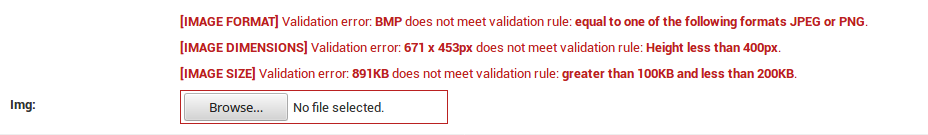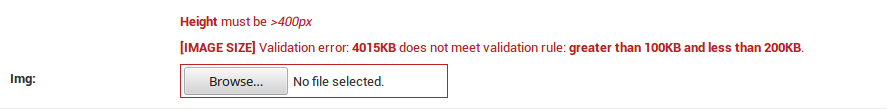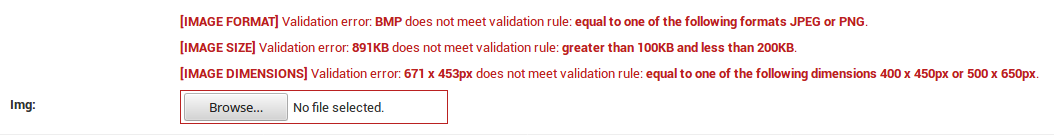# Examples¶

The following examples try to cover a variety of `VIMAGE` usages by combining different specificity values and showing their effect on the appropriate fields.

## With specificity 1¶

The validation rule below will be applied to all `ImageField`’s of the `my_app` app. Each image should be less than 120KB and have aspect ratio equal to 1.2.

```VIMAGE = {
'my_app.models': {
'SIZE': {
'lt': 120,
}
'ASPECT_RATIO': 1.2,
},
}
```

If we try to upload an image which is more than 120KB and it’s aspect ratio is not 1.2 then we’ll get the following default error:Since `'SIZE'` is a `dict` we can define an `'err'` key and give it a custom error:

```VIMAGE = {
'my_app.models': {
'SIZE': {
'lt': 120,
'err': 'Wrong size. Must be < 120KB',
}
'ASPECT_RATIO': 1.2,
},
}
```

which yields:## With specificity 2¶

The validation rule below will be applied to all `ImageField`’s of the `MyModel` model. Each image should be a JPEG image, equal to 400 x 500px and less than 200KB.

```VIMAGE = {
'my_app.models.MyModel': {
'FORMAT': 'jpeg',
'DIMENSIONS': (400, 500),
'SIZE': {
'lt': 200,
},
},
}
```

## With specificity 3¶

The validation rule below will be applied only to the `img` `ImageField` field. It should be a JPEG or a PNG image, the height should be less than 400px and be greater than 100KB but less than 200KB.

```VIMAGE = {
'my_app.models.MyModel.img': {
'FORMAT': ['jpeg', 'png'],
'DIMENSIONS': {
'h': {
'lt': 400,
}
},
'SIZE': {
'gt': 100,
'lt': 200,
},
},
}
```

Trying to save the object with an invalid image, we get the following default error:A custom error on `'h'` (height) may be declared, as follows:

```VIMAGE = {
'my_app.models.MyModel': {
'FORMAT': ['jpeg', 'png'],
'DIMENSIONS': {
'h': {
'lt': 400,
'err': '<strong>Height</strong> must be <em>>400px</em>',
}
},
'SIZE': {
'gt': 100,
'lt': 200,
},
},
}
```

Trying with an invalid image, we get (note that we have provided a valid image format, so the `'FORMAT'` validation passes and not shown):## With specificity 1 + 2¶

```VIMAGE = {
# specificity 1
'my_app.models': {
'FORMAT': ['jpeg', 'png'],
'SIZE': {
'gt': 100,
'lt': 200,
},
},
# specificity 2
'my_app.models.ModelOne': {
'DIMENSIONS': [(400, 450), (500, 650)],
},
# specificity 2
'my_app.models.ModelTwo': {
'FORMAT': ['webp'],
},
}
```

After declaring the above validation rule, the following rules will apply:

all `ImageField`’s of the Rules
`ModelOne` model
• `'FORMAT': ['jpeg', 'png']`
• `'SIZE': {'gt': 100, 'lt': 200}`
• `'DIMENSIONS': [(400, 450), (500, 650)]`
`ModelTwo` model
• `'FORMAT': ['webp']`
• `'SIZE': {'gt': 100, 'lt': 200}`

and providing (again) an invalid image, we get the following default error for the `img` `ImageField` inside the `ModelOne` model:## With specificity 1 + 3¶

```VIMAGE = {
# specificity 1
'my_app.models': {
'DIMENSIONS': {
'lte': (1920, 1080),
},
'FORMAT': 'jpeg',
'SIZE': {
'gt': 100,
'lt': 200,
},
},
# specificity 3
'my_app.models.ModelOne.img': {
'DIMENSIONS': (800, 1020),
},
}
```

After declaring the above validation rule, the following rules will apply:

Fields Rules
all `ImageField`’s of the `my_app` app
• `'DIMENSIONS': {'lte': (1920, 1080)}`
• `'FORMAT': 'jpeg'`
• `'SIZE': {'gt': 100, 'lt': 200}`
only the `img` field
• `'DIMENSIONS': (800, 1020)`
• `'FORMAT': 'jpeg'`
• `'SIZE': {'gt': 100, 'lt': 200}`